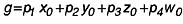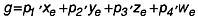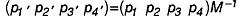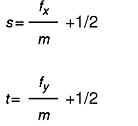[ Previous | Next | Contents | Glossary | Home | Search ]
OpenGL 1.1 for AIX: Reference Manual

## glTexGen Subroutine

### Purpose

Controls the generation of texture coordinates.

### Library

OpenGL C bindings library: libGL.a

### C Syntax

```void glTexGend(GLenum Coordinate,
GLenum pName,
GLdouble Parameter)```
```void glTexGenf(GLenum Coordinate,
GLenum pName,
GLfloat Parameter)```
```void glTexGeni(GLenum Coordinate,
GLenum pName,
GLint Parameter)```
```void glTexGendv(GLenum Coordinate,
GLenum pName,
const GLdouble *Parameters)```
```void glTexGenfv(GLenum Coordinate,
GLenum pName,
const GLfloat *Parameters)```
```void glTexGeniv(GLenum Coordinate,
GLenum pName,
const GLint *Parameters)```

### Parameters

#### glTexGend, glTexGenf or glTexGeni

 Coordinate Specifies a texture coordinate. Must be one of the following: GL_S GL_T GL_R GL_Q pName Specifies the symbolic name of the texture-coordinate generation function. Must be GL_TEXTURE_GEN_MODE. Parameter Specifies a single-valued texture generation parameter, one of GL_OBJECT_LINEAR, GL_EYE_LINEAR, or GL_SPHERE_MAP.

#### glTexGendv, glTexGenfv or glTexGeniv

 Coordinate Specifies a texture coordinate. Must be one of the following: GL_S GL_T GL_R GL_Q pName Specifies the symbolic name of the texture-coordinate generation function or function parameters. Must be GL_TEXTURE_GEN_MODE, GL_OBJECT_PLANE, or GL_EYE_PLANE. Parameters Specifies a pointer to an array of texture generation parameters. If pName is GL_TEXTURE_GEN_MODE, the array must contain a single symbolic constant, one of GL_OBJECT_LINEAR, GL_EYE_LINEAR, or GL_SPHERE_MAP. Otherwise, Parameters holds the coefficients for the texture-coordinate generation function specified by pName.

### Description

The glTexGen subroutine selects a texture-coordinate generation function or supplies coefficients for one of the functions. The Coordinate parameter names one of the (s, t, r, q) texture coordinates, and it must be one of these symbols: GL_S, GL_T, GL_R, or GL_Q. The pName parameter must be one of three symbolic constants: GL_TEXTURE_GEN_MODE, GL_OBJECT_PLANE, or GL_EYE_PLANE. If pName is GL_TEXTURE_GEN_MODE, the Parameters parameter chooses a mode, one of GL_OBJECT_LINEAR, GL_EYE_LINEAR, or GL_SPHERE_MAP. If pName is either GL_OBJECT_PLANE or GL_EYE_PLANE, the Parameters parameter contains coefficients for the corresponding texture generation function.

If the texture generation function is GL_OBJECT_LINEAR, the following function is used:where g is the value computed for the coordinate named in the Coordinate parameter, p1, p2, p3, and p4 are the four values supplied in the Parameters parameter, and x0, y0, z0, w0 are the object coordinates of the vertex. This function can be used to texture-map terrain using sea level as a reference plane (defined by p1, p2, p3, and p4). The altitude of a terrain vertex is computed by the GL_OBJECT_LINEAR coordinate generation function as its distance from sea level; that altitude is used to index the texture image to map white snow onto peaks and green grass onto foothills, for example.

If the texture generation function is GL_EYE_LINEAR, the following function is used:where:and xe, ye, ze, and we are the eye coordinates of the vertex, p1, p2, p3, p4 are the values supplied in Parameters, and M is the modelview matrix when glTexGen is invoked. If M is poorly conditioned or singular, texture coordinates generated by the resulting function may be inaccurate or undefined.

Note that the values in the Parameters parameter define a reference plane in eye coordinates. The modelview matrix that is applied to them may not be the same one in effect when the polygon vertices are transformed. This function establishes a field of texture coordinates that can produce dynamic contour lines on moving objects.

If the pName parameter is GL_SPHERE_MAP and the Coordinate parameter is either GL_R or GL_Q, s and t texture coordinates are generated as follows. Let u be the unit vector pointing from the origin to the polygon vertex (in eye coordinates). Let n´ be the current normal, after transformation to eye coordinates. Let f=(fx fy fz)T be the reflection vector such that

`f=u-2n´n´Tu`

Finally, let m=2(square root (fx2+fy2+(fz+1)2)). Then the values assigned to the s and t texture coordinates are the following:A texture-coordinate generation function is enabled or disabled using the glEnable or glDisable subroutines with one of the symbolic texture-coordinate names (GL_TEXTURE_GEN_S, GL_TEXTURE_GEN_T, GL_TEXTURE_GEN_R, or GL_TEXTURE_GEN_Q) as the argument. When enabled, the specified texture coordinate is computed according to the generating function associated with that coordinate. When disabled, subsequent vertices take the specified texture coordinate from the current set of texture coordinates. Initially, all texture generation functions are set to GL_EYE_LINEAR and are disabled. Both s plane equations are (1,0,0,0), both t plane equations are (0,1,0,0), and all r and q plane equations are (0,0,0,0).

### Error Codes

 GL_INVALID_ENUM Coordinate or pName is not an accepted defined value, or pName is GL_TEXTURE_GEN_MODE and Parameters is not an accepted defined value. GL_INVALID_ENUM pName is GL_TEXTURE_GEN_MODE, Parameters is GL_SPHERE_MAP, and Coordinate is either GL_R or GL_Q. GL_INVALID_OPERATION The glTexGen subroutine is called between a call to glBegin and the corresponding call to glEnd.

### Associated Gets

Associated gets for the glTexGen subroutine are as follows. (See the glGet subroutine for more information.)

glGetTexGen

glIsEnabled with argument GL_TEXTURE_GEN_S

glIsEnabled with argument GL_TEXTURE_GEN_T

glIsEnabled with argument GL_TEXTURE_GEN_R

glIsEnabled with argument GL_TEXTURE_GEN_Q.

### Files

 /usr/include/GL/gl.h Contains C language constants, variable type definitions, and ANSI function prototypes for OpenGL.

### Related Information

The glBegin or glEnd subroutine, glEnable or glDisable subroutine, glGetTexGen subroutine, glTexEnv subroutine, glTexImage1D subroutine, glTexImage2D subroutine, glTexImage3DEXT subroutine, glTexParameter subroutine.

[ Previous | Next | Contents | Glossary | Home | Search ]